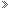Log in (Register | Forgotten password)You are not registered or have not logged in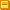Hello guest! (Not a guest? Log in above!) As a guest on TeenHelp you are only able to use some of our site's features. By registering an account you will be able to enjoy unlimited access to our site, and will be able to: Connect with thousands of teenagers worldwide by actively taking part in our Support Forums and Chat Room. Find others with similar interests in our Social Groups. Express yourself through our Blogs, Picture Albums and User Profiles. And much much more! Signing up is free, anonymous and will only take a few moments, so click here to register now!

Education and Careers Work of any kind can get stressful at times. Ask in this forum if you need help with coursework, applications, and more.

 Brandon Offline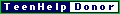Member I can't get enough*********   Name: Brandon Age: 30 Gender: Male Posts: 2,542 Join Date: January 6th 2009 College Algebra Questions - August 21st 2009, 08:03 PM I just got done with my first week of college, and I'm in a College Algebra math class. To finish off the week, the teacher gave us some work to do over the weekend, and I have a bunch of questions. Right now we're doing functions. So if this is something that you know well and can explain in a simplified manner, then that'd be a great help. The first problem that I'm having trouble with is this: f(x)= ((4x^2+1)/(x^3)) a. 2 b. -2 c. -x The objective of the problems are to "evaluate each function at the given values of the independent variable and simplify". For f(2) and f(-2), I came up with 15/4. For (-x), I came up with 3x-1...not confident in that answer at all. My second problem is in a graph, which I made here: http://img522.imageshack.us/img522/8898/graph1.png The x-intercepts are 1 and -3 and the y-intercept is -3. The bottom of the function starts at -4, just clarifying if that graph is too sloppy to understand. What I need to figure out is: a. the function's domain b. the function's range c. the x-intercepts d. the y-intercepts e. and the missing function values that are indicated by question marks: f(-2) = ? and f(2) = ? For the domain, I put {x|-3≤x≤1}, and for the range I put {y|y≥-4}, but I'm not confident in those answers. I don't understand e period, so how would I find the missing function values? The last question that I have for now is another graph that has me completely stumped. It looks like this: http://img32.imageshack.us/img32/2284/graph1k.png Again, I have to find the domain, range, x-intercept and y-intercept, and the missing function values that are indicated with a question mark from f(-1) = ? and f(3) = ? I'd appreciate your help VERY MUCH.
 <:3 )~ Offline squeak Average Joe***   Name: olivia Age: 28 Gender: Female Location: Across the Universe Posts: 195 Blog Entries: 2 Join Date: May 26th 2009 Re: College Algebra Questions - August 21st 2009, 08:20 PM I'll take a stab at it... f(x)=(4(2)²+1)/(2³) =(4*4+1)/8 ---> 2*2*2=8 =(16+1)/8 =17/8 f(x)= (4(-2)²+1)/-2³ = (4*4+1)/-8 =-17/8 f(x)=4(-x)²+1/(-x)³ =4x+1/-x = -4x+1/x On the first picture: I'm sorry. I just read what the roots were (x-intercepts) from this we get the equation (x-1)(x+3)=x²+2x-3 which is the parabola's equation. The domain (x-values) is...x has to be greater than or equal to -3 and and less than or equal to 1. x>-3 and x<1 1>x>3 is the domain {x|1>x>3} The range is y>3 {y|y>3} This second graph looks like an absolute value graph. The parent function for an absolute value is y=|x|. Say it's x=-1. y=|1| and y=|-1|. This results in a mirror image across the y-axis. So if you fold the graph it's identical on both sides. The equation of this graph is roughly y=|x|+1 (as it's shifted up one from the parent function with a vertex at 0,0) To find out the x-intercept look at the graph. Where does it intercept the x-axis? Nowhere. So there is no x-intercept. The y-intercept: Where does it intersect on the y-axis? That would be at (0,1) as you can see there. =) The domain is just where the x-values begin and where they end (in layman's terms). So, the x values begin at x>0 and continue on for infinity. So, x>0 is the domain. And in set notation: {x|x>0} The range is just where the y-values being and where they end. Well, the only point where there is even a valid y point is at y=1. So the range is y> 1 (as you can see, the values continue on from 1 to foreverrr thanks to that arrow) In set notation: {y|y>1} For the missing values you can refer to the picture OR plug and chug. I'll plug and chug. =) f(x)=|x|+1 =|-1|+1 =1+1 =2 f(x)=|3|+1 =|3|+1 =3+1 =4 I hope I could help and I apologize for any mistakes as I'm a little math rusty from the summer. PM me if you need any more help. =) Live and let live.Last edited by <:3 )~; August 21st 2009 at 08:50 PM.
Brandon Offline
Member
I can't get enough
*********

Name: Brandon
Age: 30
Gender: Male

Posts: 2,542
Join Date: January 6th 2009

Re: College Algebra Questions - August 21st 2009, 09:47 PM

Quote:
 For the missing values you can refer to the picture OR plug and chug. I'll plug and chug. =) f(x)=|x|+1 =|-1|+1 =1+1 =2 f(x)=|3|+1 =|3|+1 =3+1 =4
Where did you get the -1 and the 3 in the absolute value signs? And how could I look at the picture and determine what replaces the question mark?

 <:3 )~ Offline squeak Average Joe***   Name: olivia Age: 28 Gender: Female Location: Across the Universe Posts: 195 Blog Entries: 2 Join Date: May 26th 2009 Re: College Algebra Questions - August 21st 2009, 09:48 PM Because if you slide over -3 on the x-axis and see what lies above it in y-axis terms, that would be your y value. Well, x=-1, x=3. That's the equation for the function so just plug them in and solve for y. =) Live and let live.Brandon Offline
Member
I can't get enough
*********

Name: Brandon
Age: 30
Gender: Male

Posts: 2,542
Join Date: January 6th 2009

Re: College Algebra Questions - August 21st 2009, 11:10 PM

Quote:
 Because if you slide over -3 on the x-axis and see what lies above it in y-axis terms, that would be your y value. Well, x=-1, x=3. That's the equation for the function so just plug them in and solve for y. =)
Sorry, I still don't quite understand.

Quote:
 f(-2) = ? and f(2) = ?
For e, I have to find the missing values of the function f(-2) and f(2). Don't the -2 and 2 mean something because each graph problem, they have different numbers in the f(number).

 <:3 )~ Offline squeak Average Joe***   Name: olivia Age: 28 Gender: Female Location: Across the Universe Posts: 195 Blog Entries: 2 Join Date: May 26th 2009 Re: College Algebra Questions - August 22nd 2009, 02:45 AM Oh okay. To find -2 and 2 plug and chug. Plug in the number and solve! f(x)=|x|+1 x=-2 f(x)=|-2|+1 =2+1 =3 f(x)=|x|+1 x=2 f(x)=|2|+1 f(x)=3 Basically it's showing how the absolute value symbol creates a graph that is symmetric on the y-axis. Also I would like to add this information: f(x) mean the function of x. y is a function of x. Which means it changes as x changes basically. f(x)=y Okay, on the thing you don't understand - remember plotting points? Slide over -3 on the x-axis and plot the first point you see on the function itself with a y coordinate by scaling your pencil upward vertically. Like that. =) Live and let live.Last edited by <:3 )~; August 22nd 2009 at 02:56 AM.
Brandon Offline
Member
I can't get enough
*********

Name: Brandon
Age: 30
Gender: Male

Posts: 2,542
Join Date: January 6th 2009

Re: College Algebra Questions - August 22nd 2009, 04:32 AM

Quote:
 Originally Posted by <:3 )~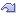Oh okay. To find -2 and 2 plug and chug. Plug in the number and solve! f(x)=|x|+1 x=-2 f(x)=|-2|+1 =2+1 =3 f(x)=|x|+1 x=2 f(x)=|2|+1 f(x)=3 Basically it's showing how the absolute value symbol creates a graph that is symmetric on the y-axis. Also I would like to add this information: f(x) mean the function of x. y is a function of x. Which means it changes as x changes basically. f(x)=y Okay, on the thing you don't understand - remember plotting points? Slide over -3 on the x-axis and plot the first point you see on the function itself with a y coordinate by scaling your pencil upward vertically. Like that. =)

I figured it out, but in a different way. I'm good from here on. Thanks for your help though.

 <:3 )~ Offline squeak Average Joe***   Name: olivia Age: 28 Gender: Female Location: Across the Universe Posts: 195 Blog Entries: 2 Join Date: May 26th 2009 Re: College Algebra Questions - August 22nd 2009, 07:09 PM No problem! I'm glad that you figured it out. It's kinda difficult to explain it in text and not be showing somebody it.Best of luck in that algebra class x Live and let live.Tags algebra, college, questionsThread Tools Search this Thread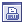Show Printable Version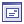Email this Page Search this Thread: Advanced Search Rate This Thread Rate This Thread: 5 : Excellent 4 : Good 3 : Average 2 : Bad 1 : TerriblePosting Rules You may post new threads You may post replies You may not post attachments You may not edit your posts BB code is On Smilies are On [IMG] code is On HTML code is OffTrackbacks are Off Pingbacks are Off Refbacks are Off Forum Rules